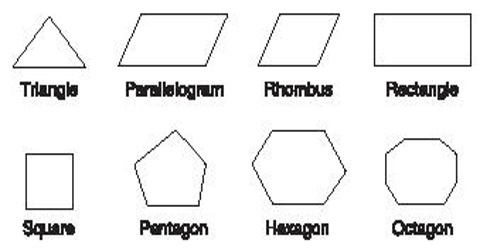### Triangles: Definition with Types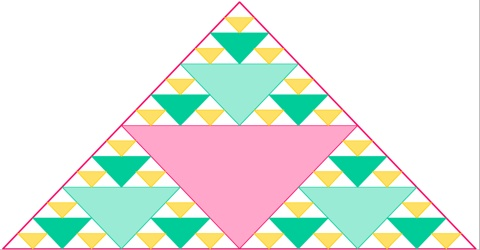Triangle is a plane figure with three straight sides and three angles. The three angles always add to 180°. The vertex is a corner of the triangle. Every triangle has three vertices. The interior angles of a triangle always add up to 180°. The exterior angles of a triangle always add up to 360�.....

### Area of a Polygon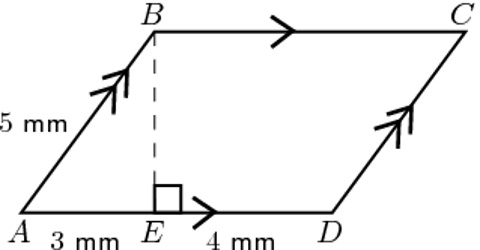Area of a Polygon A polygon is a two-dimensional shape that is bounded by line segments. It can have as many as finite number of line segments. Calculating the area of a polygon can be as easy as finding the area of a regular triangle or as complicated as finding the area of an irregular [&hellip.....

### Dodecagon Polygon: Definition, Types and PropertiesDodecagon: Dodecagon is one of the types of polygon which has twelve sides. There is an important type of polygon which is known as dodecagon. It is a polygon which is bounded by twelve line segments. More specifically, a dodecagon has 12 sides (or edges) and 12 internal (or interior) angles. The.....

### Decagon Polygon: Definition, Types and properties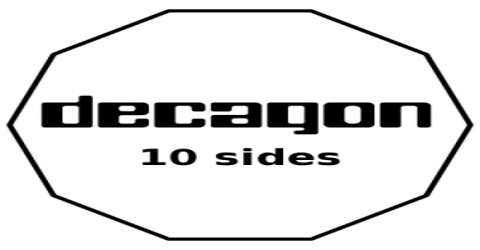A decagon is defined as a polygon with ten sides. The word “deca” itself means “ten”. A decagon has 10 sides and 10 internal angles. It has ten vertices as well. Decagons may be irregular or regular. Properties of Decagon Each angle in a regular decagon is equal to 144°. .....

### Nonagon Polygon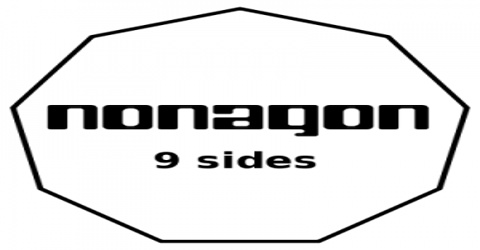Nonagon is one of the types of polygonal shapes. The nonagon is said to be a 9 sided polygon. It is also called Enneagon. The polygon sides are always straight and it is said to be a two dimensional shape. Properties of regular nonagon: The interior angle of the regular polygon is 140 degree. If .....

### Octagon Polygon: Overview and TypesOctagon is a polygon which has 8 sides is known as an octagon. It is a two-dimensional closed shape. Octagons must have straight sides that connect; they cannot be curved or disconnected. The word “octagon” is made up of octa + gonia which are Greek words. Octa means eight and gonia i.....

### Heptagon Polygon: Definition with Types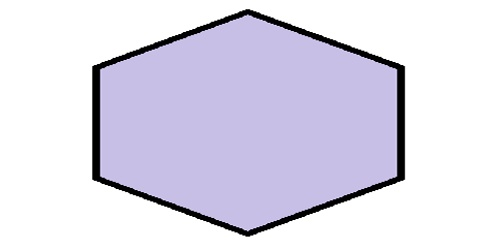Heptagon is a type of polygon that is composed of 7 lines bounded together. It is a two-dimensional figure. Therefore, a heptagon has seven sides as well as seven angles. The angles are always found at the vertices. It is also called septagon, but heptagon is more popular name. Sum of Interior An.....

### Hexagon Polygon: Overview with Types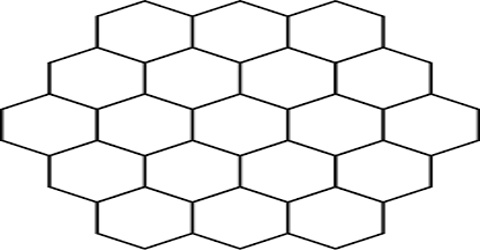Hexagon is a six-sided polygon (a flat shape with straight sides). It is a polygon of six angles and six sides. The total of the internal angles of any hexagon is 720°. A hexagon does have 6 vertices, 6 interior angles and 6 sides. Hexagons are found in many other parts of nature: the bond-shape.....

### Pentagon: Definition with Types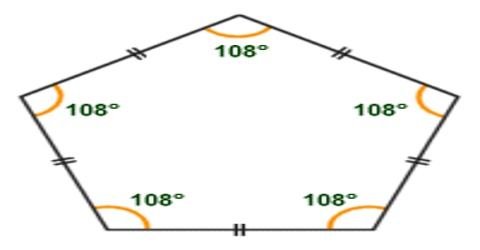A pentagon is a 5-sided Polygon. A regular pentagon has five lines of reflectional symmetry, and rotational symmetry of order 5 (through 72°, 144°, 216° and 288°). A convex pentagon has no angles pointing inwards. More precisely, no internal angles can be more than 180°. When any internal an.....A quadrilateral is a closed figure with four straight sides. Each point where two sides touch is called a vertex. Simple quadrilaterals are either convex or concave. Properties Four sides (edges) Four vertices (corners) The interior angles add up to 360 degrees Types of Quadrilaterals There are .....

### Triangle: Definition with Types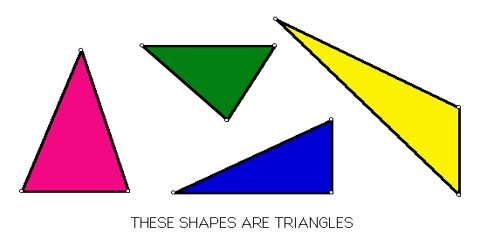A triangle has three sides and three angles. The three angles always add to 180° Every triangle has three sides and three angles, several of which may be similar. The sides of a triangle are given particular names in the case of a right triangle, with the side opposite the right angle being term.....